## Flow Network

The flow Network according to the Graph Theory, is a Directed Graph in which each edge has a non-negative capacity and each edge receives the flow. The most important feature of this graph is that the flow on an edge cannot exceed the capacity in any condition.  It is necessary that the amount of flow in a node must be equal to the flow going out of the node. This condition can violate only for source node.

## Ford Fulkerson Algorithm

The Ford Fulkerson Algorithm is an important algorithm in Flow Control developed by L.R Ford and D.R Fulkerson in 1956. It is also known as greedy algorithm as it computes the maximum flow of the directed graph. The basic concept behind this algorithm is if there is a path from source node (s) to destination or sink node (t) with capacity then another path can also be computed.

### Algorithm

There is given a Graph G (V, E) with every edge has a capacity with fulfilling following constraint-

1.The flow on an edge cannot exceed the capacity of the edge.

2.The incoming flow on a vertex must be equal to the outgoing flow of the vertex except for s and t.

### STEPS-

1. First develop the Residual Graph

2.Find the path from source to the sink with positive flow.

3.If the path exists then update flow and go to step 1.

4.Otherwise the flow is maximal.

5.The (S-T) Cut has all the vertices covered.

Capacity Constraints-  (u,v)∈Ef(u,v)≤c(u,v)       The flow cannot exceed capacity.

### Pseudo Code

Input – Network (N, s, t) with capacity function C, Flow function F

Output – Maximal flow tree with Augmented Flow function

Step1 – check for maximum flow set max flow=0

Step2 While max flow =0 so max saturated tree = t

Step3 – Find another unique path from s to t.

Step 4 – Calculate Augmented function of it.

### Ford Fulkerson Algorithm-Min Cut Problem

Problem – A cut is a partition (S, T) in a node with condition-:

Capacity (S, T) = Sum of weight of the edges leaving S (initial vertex)

The problem is finding path from S-T with minimum weight.

IMPLEMENTATION

Directed Graph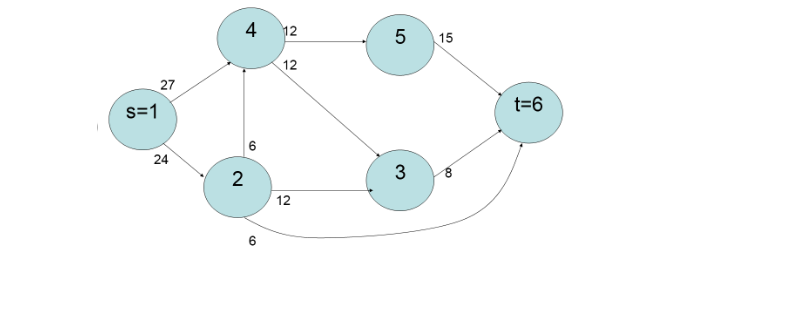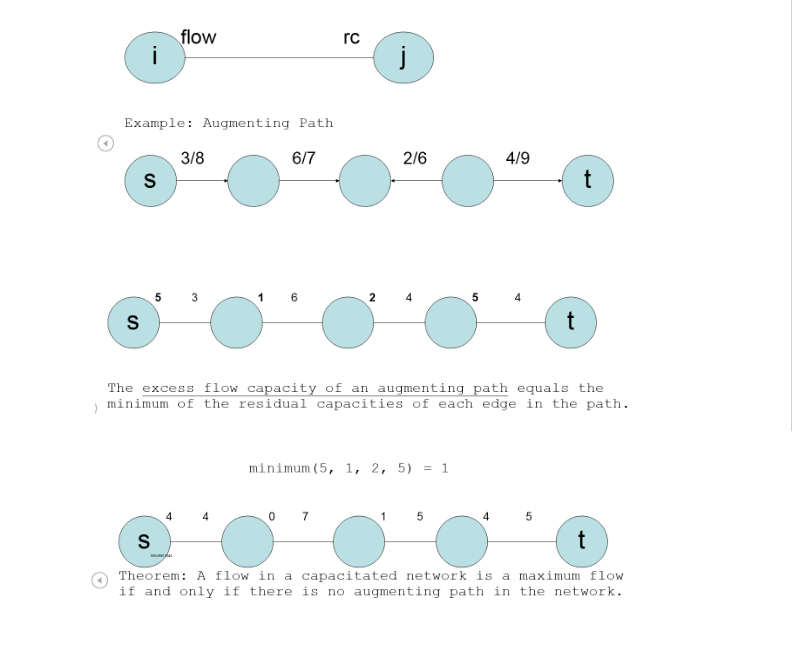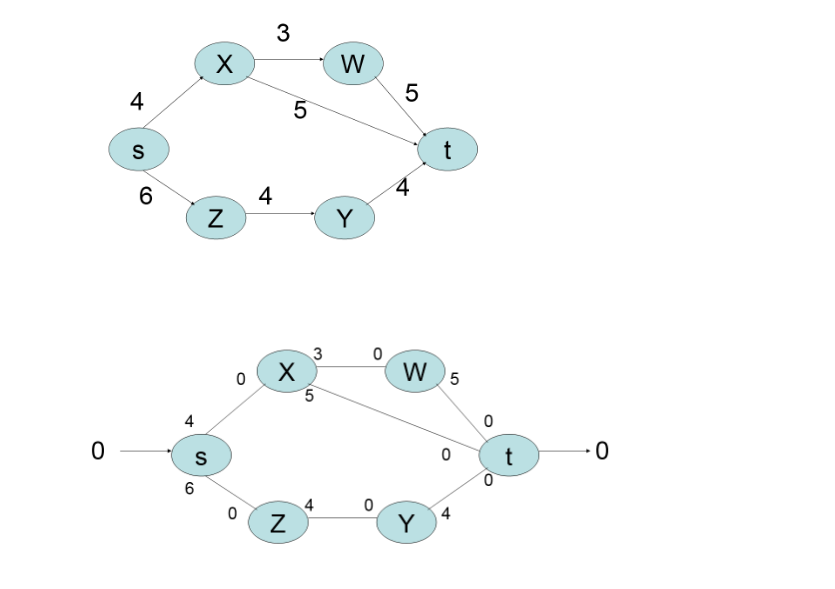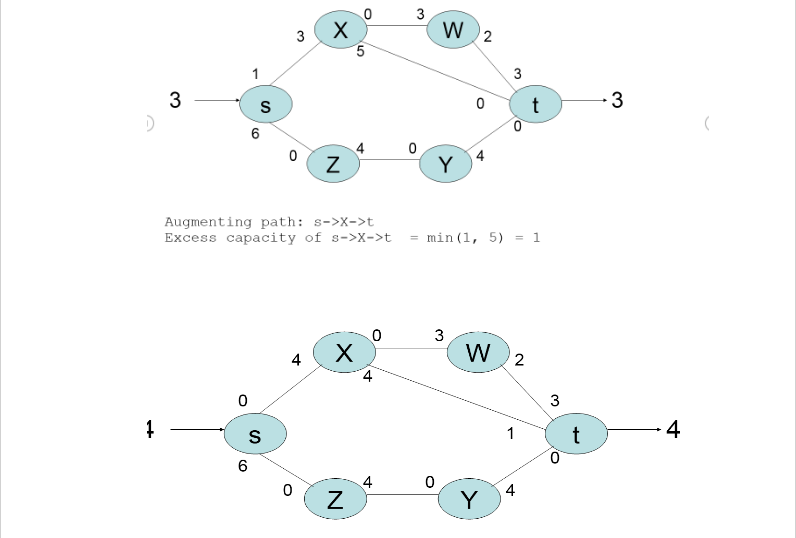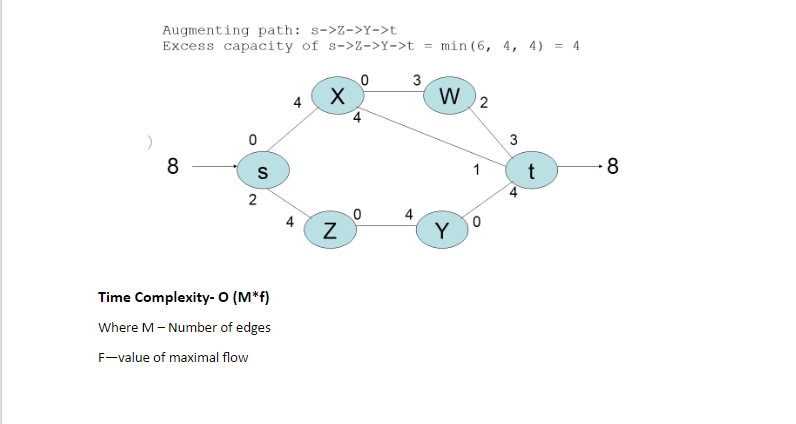Essaycorp provides data-structure and algorithm assigment help to students , which enable them to score better in academics by improving overall study experience. Timely help from Essaycorp give them additional confidence, improved interests and better performance in midterm and final exams.

Author:
Category :
Publish Date :
Social Sharing